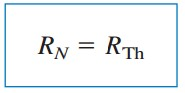omG8HI9LSKFW6lhCj8prTs0Z6lUhdOT9Jhi1Sf4m

# Norton's Theorem

In 1926, about 43 years after Thevenin published his theorem, E.L. Norton, an American engineer at Bell Telephone, proposed a similar theorem.

This circuit analysis theorems are classified as:

## Norton's Theorem Theory

Before learning about Norton's theorem, let us read the brief explanatin below :
Norton's theorem states that a linear two-terminal circuit can be replaced by an equivalent circuit consisting of a current source IN in parallel with a resistor RN, where IN is the short-circuit current through the terminals and RN is the input or equivalent resistance at the terminals when the independent sources are turned off.
Thus, the circuit in Figure.(1a) can be replaced by the one in Figure.(1b).Figure 1
We find RN in the same way we find RTh. In fact, from what we know about source transformation, the Thevenin and Norton resistances are equal; that is,(1)
To find the Norton current IN, we determine the short-circuit current flowing from terminal a to b in both circuits in Figure.(1). It is evident that the short-circuit current in Figure.(1b) is IN. This must be the same short-circuit current from terminal a to b in Figure.(1a), since the two circuits are equivalent. Thus,(2)
shown in Figure.(2). Dependent and independent sources are treated the same way as in Thevenin's theorem.Figure 2
Observe the close relationship between Norton's and Thevenin's theorems : RN = RTh as in Equation.(1), and(3)
This is essentially source transformation. For this reason, source transformation is often called Thevenin-Norton transformation.

Since VTh, IN, and RTh are related according to Equation.(3), to determine the Thevenin or Norton equivalent circuit requires that we find :
1. The open-circuit voltage voc across terminals a and b.
2. The short-circuit current isc at terminals a and b.
3. The equivalent or input resistance Rin at terminals a and b when all independent sources are turned off.
We can calculate any two of the three using the method that takes the least effort and use them to get the third using Ohm's law. Also, since(4a)(4b)(4)
the open-circuit and short-circuit test are sufficient to find any Thevenin or Norton equivalent, of a circuit which contains at least one independent source.

## Norton's Theorem Examples

For better understanding let us review the examples below :
1. Find the Norton equivalent circuit of the circuit in Figure.(3) at terminals a-b.Figure 3
Solution :
We find RN in the same way we find RTh in the Thevenin equivalent circuit. Set the independent sources equal to zero. This leads to the circuit in Figure.(4a), from which we find RN. Thus,
To find IN, we short-circuit terminals a and b, as shown in Figure.(4b). We ignore the 5 Ω resistor because it has been short circuited. Applying mesh analysis, we obtain
From these equations, we obtainFigure 4
Alternatively, we may determine IN from VTh/RTh. We obtain VTh as the open-circuit voltage across terminals a and b in Figure.(4c). Using mesh analysis, we obtain
and
Hence,
as obtained previously. This also serves to confirm Equation.(4c) that RTh = voc/isc = 4/1 = 4 Ω. Thus, the Norton equivalent circuit is as shown in Figure.(5).Figure 5

2.Using Norton's theorem, find RN and of the cicuit in Figure.(6) at terminals a-b.Figure 6
Solution :
To find RN, we set the independent voltage source equal to zero and connect a voltage source of vo = 1 V (or any unspecified voltage vo) to the terminals. We obtain the circuit in Figure.(7a). We ignore the 4 Ω resistor because it is short-circuited. Also due to the short circuit, the 5 Ω resistor, the voltage source, and the dependent current source are all in parallel. Hence ix = 0. At node a, io = 1v/5Ω = 0.2 A, and
To find IN, we short-circuited terminals a and b and find the current isc, as indicated in Figure.(7b). Note from this figure that the 4 Ω resistor, the 10 V voltage source, the 5 Ω resistor, and the dependent current source are all in parallel. Hence,
At node a, KCL gives
ThusFigure 7

Untuk Bahasa Indonesia baca Teorema Norton.
Related Posts
SHARE